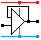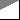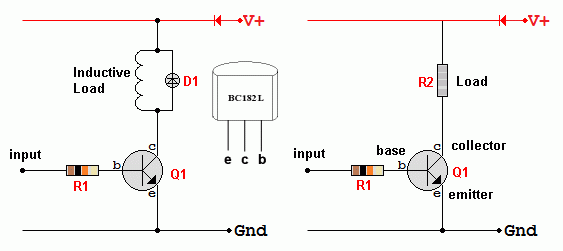Home

rOm
Quest
Glossary

Random
Page
Search
Site
Lush
Sim
Class
Subject
Images

Help
FAQ
Sign
Up
Log
In
 GCSE     Switch     >BJT<     Comparator     Fuse     Logic     Microswitch     MOSFET     Pull Up or Down     RCD     Reed     Relay     Tilt     Touch

# Switch BJT

Site for Eduqas/WJEC - Go to the AQA site.

GCSE    SwitchesQuestions 0 to 23   -->  View All

## Compare Switch Types

• BJT switches are fast and the transistors are not expensive. A minus point is the current needed to operate the switch.
• MOSFETS are even faster but they cost more. A plus point is the minute current needed to operate the switch - nearly zero.
• OP-Amp comparators are slow, more expensive, but much more sensitive to input voltage changes.
• Logic GATE switches are fast and cheap but not very sensitive to small input voltage changes.
• Mechanical switches are less reliable due to the moving parts.
• Electromagnetic relays are less reliable due to the moving parts.
• Mechanical switches and relays can switch high voltages and currents and also alternating current.

A small base current is used to switch a much larger collector current.

## Bipolar Junction Transistor Switches - OK for Low Power

• Here is a switch or transducer driver based on an NPN bipolar junction transistor.
• This is also known as a common emitter switch.
• A small base (b) current controls a much larger collector (c) current supplying the transducer.
• A high logic level in the input will switch on the bipolar junction transistor.

• To calculateR1        R1   <=   RL   x   hFE        R1 should be less than or equal to the current gain times the load resistance.

• R1 limits the base current to a few milliamps
• Q1 is an NPN transistor. For example a BC182L with a current gain of about 300
• R2 is the load. This could be a bulb, an LED, a relay, a small motor, a buzzer and so on.
• D1 is only needed if the load is inductive. Inductive loads are electromagnetic devices such as motors, relays and some buzzers.
When the current through an inductor is turned off suddenly, a large Back EMF is produced.
This could be enough to destroy the transistor.
By adding the diode, the current through the inductor can die away gradually (by flowing through the diode).
This prevents the large back EMF.

• When the transistor is saturated (fully turned on), the collector current depends only on the load resistance and the power supply voltage.
• When the transistor is cut off (fully turned off), Vce = Vs.• If VIN is less than 0.7 V
• VCE is equal to the power supply voltage.
• VBE is equal to VIN.
• The potential difference across the load is zero.
• If VIN is more than 0.7 V
• VBE is equal to 0.7V.
• VCE is approximately zero.
• The potential difference across the load is approximately equal to the power supply voltage.

• For efficient switching, the transistor should be saturated.
• When saturated, increasing the base current has no effect on the collector current.
• If the base current is too small and the transistor is not saturated and   IC   =   hFE IB
• hFE is the current gain of the transistor.

### Current Gain

• Current Gain    hFE = Ic / Ib        Values from 10 to 500 are common.

## Alternative Circuits

• Use a MOSFET. This switches faster. Bigger currents can be handled. A minute input power is needed to operate the switch. The MOSFET is voltage operated. This is often an advantage.
• Use a relay. This is mechanical, slow and relatively unreliable but enormous currents can be switched. Alternating currents can be switched too.
• Use a thyristor or a triac.

## Estimating Component Values

Here is an example ...

• The load resistance is 600 Ω
• The load current is 20mA.
• The transistor current gain is at least 200.
• To get 20mA in the collector, 20/200 mA base current is needed = 0.1 mA
• In the 12 Volt circuit above, the base resistor connected to 12V would need to be ...
• R = V / I
• R = 12 / 10-4 = 120 kΩ        A 100 kΩ resistor would be OK.
• You should now notice that the base resistor is 200 times the load resistor because the current gain is 200. This gives a quick way to estimate what base resistor to use.

## Thermal Stability and Run-Away

• When a bipolar transistor heats up, its current gain increases.
• This causes the current to increase.
• This makes it hotter.
• In a poorly designed circuit, this is a vicious cycle and can lead to thermal run-away and the destruction of the transistor.
• NEGATIVE FEEDBACK helps to solve the problem.
• Increasing current is used to shut down the device, returning the current back to a safe level.

Subject Name     Level     Topic Name     Question Heading     First Name Last Name Class ID     User ID

 CloseEscapeX Q: qNum of last_q     Q ID: Question ID         Score: num correct/num attempts         Date Done

Question Text

image url

Help Text
Debug

• You can attempt a question as many times as you like.
• If you are logged in, your first attempt, each day, is logged.
• To improve your scores, come back on future days, log in and re-do the questions that caused you problems.
• In the grade book, you can delete your answers for a topic before re-doing the questions. Avoid deleting unless you intend re-doing the questions very soon.